Maths-
General
Easy

Question

# The volume of a metallic cylindrical pipe is 748 cubic.cm Its length is 14 cm., and its external radius is 9 cm. Find its thickness?Hint:

## The correct answer is: The thickness of the pipe is 8 cm.

### Explanations:Step 1 of 1:Let the internal radius of the pipe be r .Given, external radius R  = 9cmLength = height of the pipe h = 14cmWe have volume of pipe = 748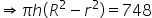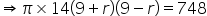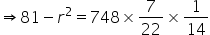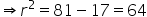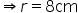Final Answer:The thickness of the pipe is 8 cm.#### With Turito Foundation.#### Get an Expert Advice From Turito.